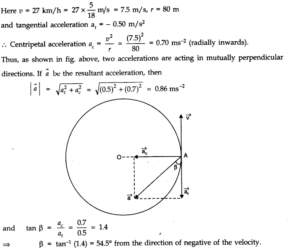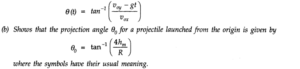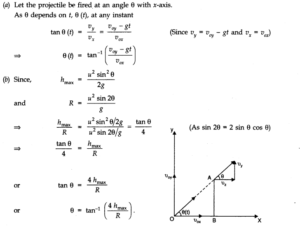# Chapter 4 – Motion in a plane Questions and Answers: NCERT Solutions for Class 11 Physics

Class 11 Physics NCERT book solutions for Chapter 4 - Motion in a plane Questions and Answers.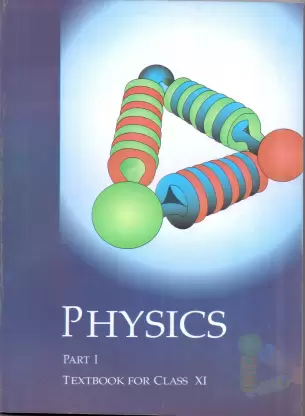## Question 4. 6. Establish the following inequalities geometrically or otherwise: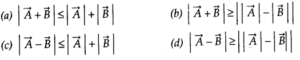## When does the equality sign above apply?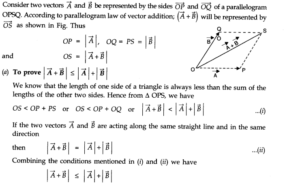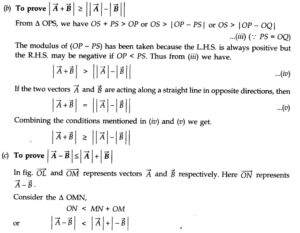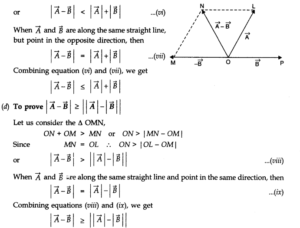## Question 4. 7.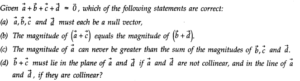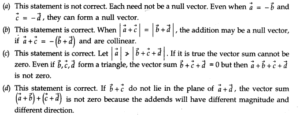## Question 4. 8. Three girls skating on a circular ice ground of radius 200 m start from a point P on the edge of the ground and reach a point Q diametrically opposite to P following different paths as shown in Fig. What is the magnitude of the displacement vector for each? For which girl is this equal to the actual length of path skate?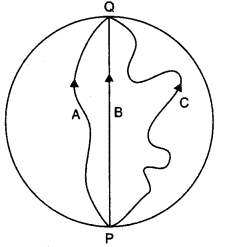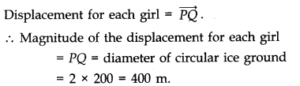## Question 4. 9. A cyclist starts from the centre O of a circular park of radius 1 km, reaches the edge P of the park, then cycles along the circumference, and returns to the centre along QO as shown in Fig. If the round trip takes 10 min, what is the (a) net displacement, (b) average velocity, and (c) average speed of the cyclist?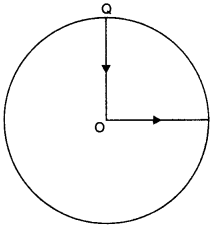### Answer:(a) Since both the initial and final positions are the same therefore the net displacement is zero. (b) Average velocity is the ratio of net displacement and total time taken. Since the net displacement is zero therefore the average velocity is also zero.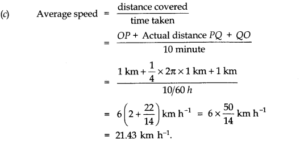## Question 4. 10. On an open ground, a motorist follows a track that turns to his left by an angle of 60° after every 500 m. Starting from a given turn, specify the displacement of the motorist at the third, sixth and eighth turn. Compare the magnitude of the displacement with the total path length covered by the motorist in each case.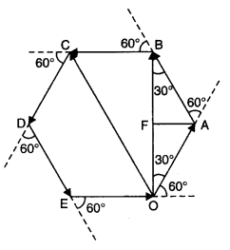### Answer:(i) The path followed by the motorist will be a closed hexagonal path. Suppose the motorist starts his journey from the , point O. He takes the turn at point C.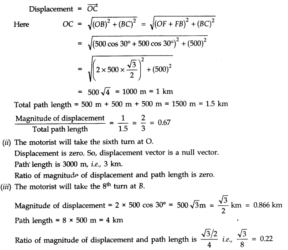## Question 4. 11.A passenger arriving in a new town wishes to go from the station to a hotel located 10 km away on a straight road from the station. A dishonest cab man takes him along a circuitous path 23 km long and reaches the hotel in 28 min. What is (a) the average speed of the taxi, (b) the magnitude of average velocity? Are the two equal?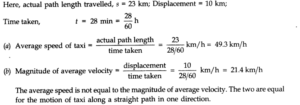## Question 4. 12. Rain is falling vertically with a speed of 30 m s-1. A woman rides a bicycle with a speed of 10 m s-1in the north to south direction. What is the direction in which she should hold her umbrella ?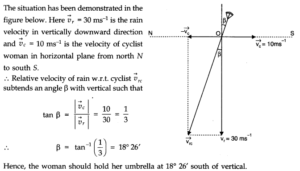## Question 4. 13. A man can swim with a speed of 4.0 km h-1in still water. How long does he take to cross a river 1.0 km wide if the river flows steadily at 3.0 km h-1and he makes his strokes normal to the river current? How far down the river does he go when he reaches the other bank?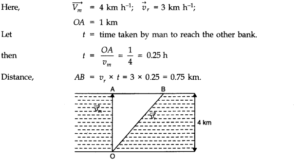## Question 4. 14. In a harbour, wind is blowing at the speed of 72 km/h and the flag on the mast of a boat anchored in the harbour flutters along the N-E direction. If the boat starts moving at a speed of 51 km/h to the north, what is the direction of the flag on the mast of the boat?

### Answer:When the boat is anchored in the harbour, the flag flutters along the N-E direction. It shows that the velocity of wind is along the north-east direction. When the boat starts moving, the flag will flutter along the direction of relative velocity of wind w.r.t. boat. Let Vwbbe the relative velocity of wind w.r.t. boat and P be the angle betweenVwband vw (see fig. below)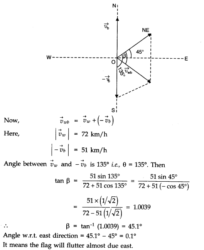## Question 4. 15. The ceiling of a long hall is 25 m high. What is the maximum horizontal distance that a ball thrown with a speed of 40 ms-1can go without hitting the ceiling of the hall?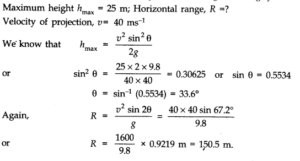## Question 4. 16. A cricketer can throw a ball to a maximum horizontal distance of 100 m. How much high above the ground can the cricketer throw the same ball?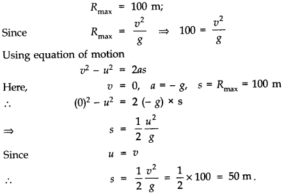## Question 4. 17. A stone tied to the end of a string 80 cm long is whirled in a horizontal circle with a constant speed. If the stone makes 14 revolutions in 25 s, what is the magnitude and direction of acceleration of the stone?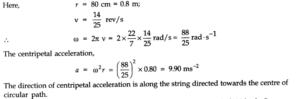## Question 4.18. An aircraft executes a horizontal loop of radius 1.00 km with a steady speed of 900 km/h. Compare its centripetal acceleration with the acceleration due to gravity.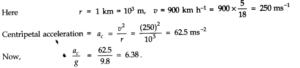## Question 4. 20. The position of a particle is given by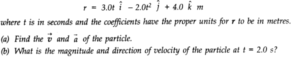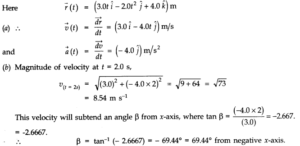## Question 4. 21.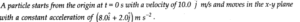## (a) At what time is the x-coordinate of the particle 16 m? What is the y-coordinate of the particle at that time? (b) What is the speed of the particle at the time?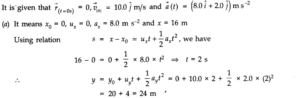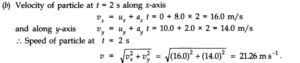## Question 4. 22.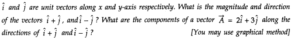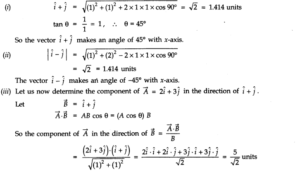##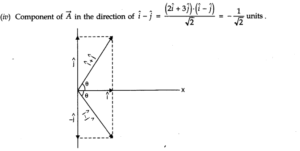Question 4. 23. For any arbitrary motion in space, which of the following relations are true: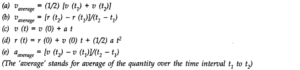## Question 4. 25. An aircraft is flying at a height of 3400 m above the ground. If the angle subtended at a ground observation point by the aircraft positions 10 s apart is 30°, what is the speed of the aircraft? Time taken by aircraft from A to B is 10 s.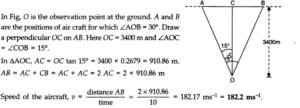## Question 4. 29. A bullet fired at an angle of 30° with the horizontal hits the ground 3 km away. By adjusting its angle of projection, can one hope to hit a target 5 km away? Assume the muzzle speed to the fixed, and neglect air resistance.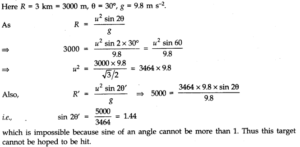## Question 4. 30. A fighter plane flying horizontally at an altitude of 1.5 km with speed 720 km h-1passes directly overhead an anti-aircraft gun. At what angle from the vertical should the gun be fired for the shell with muzzle speed 600 m s-1to hit the plane? At what minimum altitude should the pilot fly the plane to avoid being hit? (Take g = 10 m s-2)?

### Answer:Velocity of plane, vp=720 x 5/180 ms-1=200 ms-1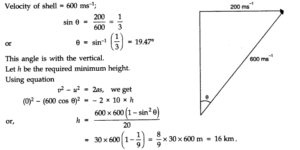## Question 4. 31. A cyclist is riding with a speed of 27 km/h. As he approaches a circular turn on the road of radius 80 m, he applies brakes and reduces his speed at the constant rate of 0.50 m/s every second. What is the magnitude and direction of the net acceleration of the cyclist on the circular turn?